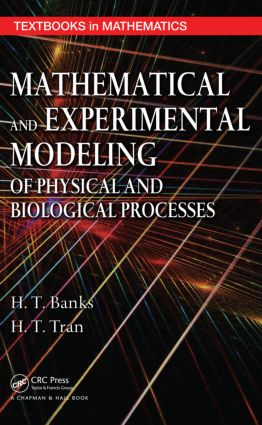# Mathematical and Experimental Modeling of Physical and Biological Processes

## 1st Edition

Chapman and Hall/CRC

298 pages | 96 B/W Illus.

##### Purchasing Options:\$ = USD
Hardback: 9781420073379
pub: 2009-01-12
SAVE ~\$22.00
\$110.00
\$88.00
x
eBook (VitalSource) : 9780429136535
pub: 2009-01-12
from \$55.00

FREE Standard Shipping!

### Description

Through several case study problems from industrial and scientific research laboratory applications, Mathematical and Experimental Modeling of Physical and Biological Processes provides students with a fundamental understanding of how mathematics is applied to problems in science and engineering. For each case study problem, the authors discuss why a model is needed and what goals can be achieved with the model.

Exploring what mathematics can reveal about applications, the book focuses on the design of appropriate experiments to validate the development of mathematical models. It guides students through the modeling process, from empirical observations and formalization of properties to model analysis and interpretation of results. The authors also describe the hardware and software tools used to design the experiments so faculty/students can duplicate them.

Integrating real-world applications into the traditional mathematics curriculum, this textbook deals with the formulation and analysis of mathematical models in science and engineering. It gives students an appreciation of the use of mathematics and encourages them to further study the applied topics. Real experimental data for projects can be downloaded from CRC Press Online.

### Reviews

…would I buy this textbook? Again, absolutely yes! The concise and clear style in which the background is written for each chapter will be invaluable as a quick, ‘before the lecture is given’ refresher. … Most of the topics covered are those which have arisen out of the research projects that the authors have conducted themselves. This is the kind of hands-on experience that a lecturer would need in order to make the laboratory experiences for the students enjoyable and rewarding. … the true value of this textbook, namely, [is that] it provides a stimulus package to provoke the reader to adopt a similar teaching philosophy.

Mathematical Reviews, Issue 2010f

The aim of this book is twofold: to develop some standard models of physical and biological processes (the transport equation, heat conduction, the beam equation, fluid dynamics, and structured population models) in mathematical language, and probably more importantly, to show how and why to design concrete engineering experiments for comparing numerical results of models with specific experimental data. … The book can be recommended to advanced undergraduate students for whom mathematics is a bit more than just proving theorems. Teachers can find suggestions for motivations for introductory parts of lectures on ordinary differential equations and partial differential equations.

EMS Newsletter, September 2009

### Table of Contents

Introduction: The Iterative Modeling Process

Modeling and Inverse Problems

Mechanical Vibrations

Inverse Problems

Mathematical and Statistical Aspects of Inverse Problems

Probability and Statistics Overview

Parameter Estimation or Inverse Problems

Computation of sigman, Standard Errors, and Confidence Intervals

Investigation of Statistical Assumptions

Statistically Based Model Comparison Techniques

Mass Balance and Mass Transport

Introduction

Compartmental Concepts

Compartment Modeling

General Mass Transport Equations

Heat Conduction

Motivating Problems

Mathematical Modeling of Heat Transfer

Experimental Modeling of Heat Transfer

Structural Modeling: Force/Moments Balance

Motivation: Control of Acoustics/Structural Interactions

Introduction to Mechanics of Elastic Solids

Deformations of Beams

Separation of Variables: Modes and Mode Shapes

Numerical Approximations: Galerkin’s Method

Energy Functional Formulation

The Finite Element Method

Experimental Beam Vibration Analysis

Beam Vibrational Control and Real-Time Implementation

Introduction

Controllability and Observability of Linear Systems

Design of State Feedback Control Systems and State Estimators

Pole Placement (Relocation) Problem

Linear Quadratic Regulator Theory

Beam Vibrational Control: Real-Time Feedback Control Implementation

Wave Propagation

Fluid Dynamics

Fluid Waves

Experimental Modeling of the Wave Equation

Size-Structured Population Models

Introduction: A Motivating Application

A Single Species Model (Malthusian Law)

The Logistic Model

A Predator/Prey Model

A Size-Structured Population Model

The Sinko–Streifer Model and Inverse Problems

Size Structure and Mosquitofish Populations

Appendix A: An Introduction to Fourier Techniques

Fourier Series

Fourier Transforms

Appendix B: Review of Vector Calculus

References appear at the end of each chapter.

### Subject Categories

##### BISAC Subject Codes/Headings:
MAT000000
MATHEMATICS / General
MAT003000
MATHEMATICS / Applied